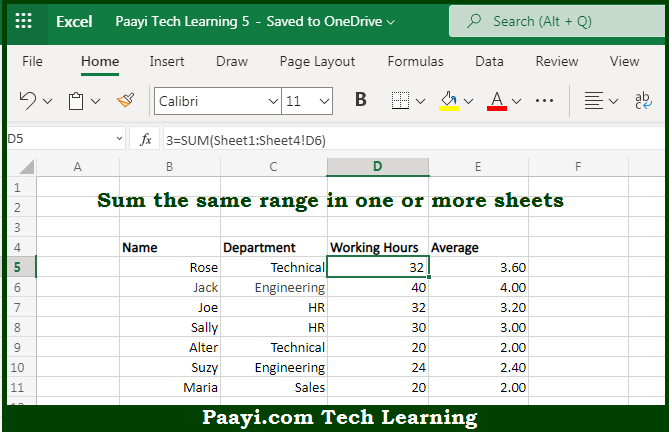# Learn How to 3D SUM Multiple Worksheets in Microsoft Excel

Written by | 0 Comments | 603 Views

In this article, you will learn how to COUNT various things in Microsoft Excel using a single/combination(s) of functions. You will also know how to 3D SUM Multiple Worksheets and see the generic formula.

3D SUM Multiple Worksheets in Microsoft Excel

The main purpose of this formula is to sum the same range in one or more sheets. Here we will learn how to generate a 3D SUM multiple worksheets in Microsoft Excel. That implies, with the help of a formula based on the SUM function with the 3D reference you can able to sum the same range in one or more sheets. So, with the help of this formula, you can able to generate a 3D SUM multiple worksheets in Microsoft Excel.

General Formula to 3D SUM Multiple Worksheets

=SUM(Sheet1:Sheet3!A1)

The Explanation for the 3D SUM Multiple WorksheetsSo we know that with the help of the given formula above you can able to sum the same range in one or more sheets. Here we will learn how to generate a 3D SUM multiple worksheets in Microsoft Excel. As we know that the syntax for referencing a range of sheets is a built-in feature and works a bit like a reference to a range of cells. It should be noted that you can use 3D references in other formulas as well So, with the help of this formula, you can able to generate a 3D SUM multiple worksheets in Microsoft Excel.